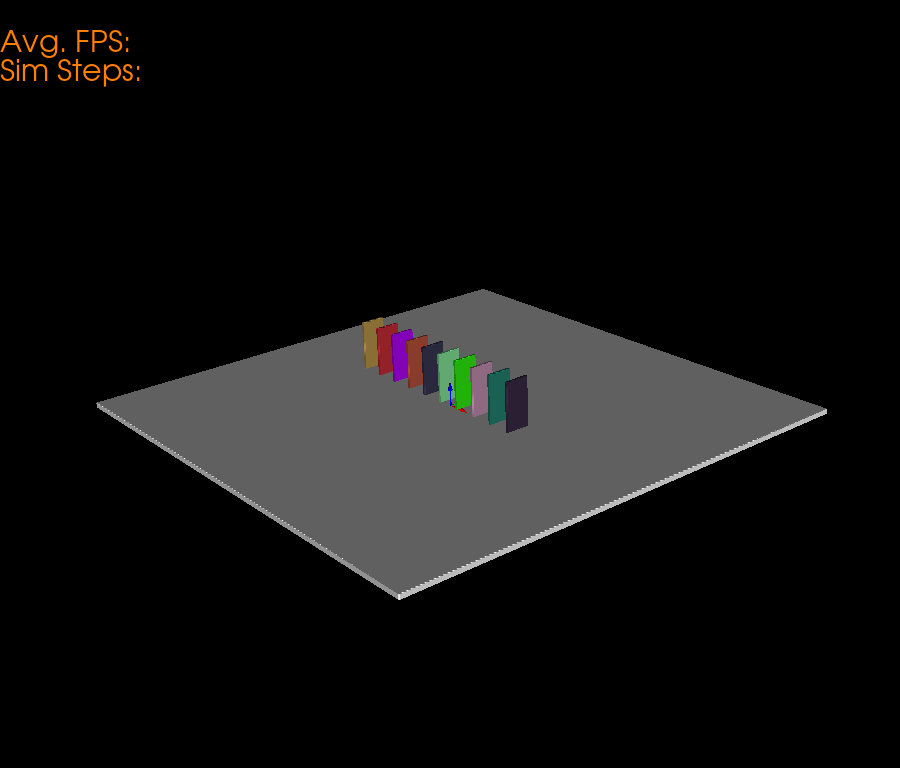# Domino Physics Simulation#

This example simulation shows how to use pybullet to render physics simulations in fury. In this example we specifically render a series of Dominoes which are under Domino Effect.

First some imports.

```import itertools

import numpy as np
import pybullet as p

from fury import actor, ui, utils, window

# Next, we initialize a pybullet client to render the physics.
# We use `DIRECT` mode to initialize pybullet without a GUI.
client = p.connect(p.DIRECT)

# Apply gravity to the scene.
p.setGravity(0, 0, -10, physicsClientId=client)
```

Set the Number of Dominoes for Simulation.

```number_of_dominoes = 10

# Base Plane Parameters
base_size = np.array([number_of_dominoes * 2, number_of_dominoes * 2, 0.2])
base_color = np.array([1, 1, 1])
base_position = np.array([0, 0, -0.1])
base_orientation = np.array([0, 0, 0, 1])

# Render a BASE plane to support the Dominoes.
base_actor = actor.box(
centers=np.array([[0, 0, 0]]),
directions=[0, 0, 0],
scales=base_size,
colors=base_color,
)

# half of the actual size.
base_coll = p.createCollisionShape(p.GEOM_BOX, halfExtents=base_size / 2)

base = p.createMultiBody(
baseCollisionShapeIndex=base_coll,
basePosition=base_position,
baseOrientation=base_orientation,
)

p.changeDynamics(base, -1, lateralFriction=1, restitution=0.5)
```

We define some global parameters of the Dominoes so that its easier for us to tweak the simulation.

```domino_mass = 0.5
domino_size = np.array([0.1, 1, 2])

domino_centers = np.zeros((number_of_dominoes, 3))

# Keeping all the dominos Parallel
domino_directions = np.zeros((number_of_dominoes, 3))
domino_directions[:] = np.array([1.57, 0, 0])

domino_orns = np.zeros((number_of_dominoes, 4))

domino_sizes = np.zeros((number_of_dominoes, 3))
domino_sizes[:] = domino_size

domino_colors = np.random.rand(number_of_dominoes, 3)

domino_coll = p.createCollisionShape(p.GEOM_BOX, halfExtents=domino_size / 2)

# We use this array to store the reference of domino objects in pybullet world.
dominos = np.zeros(number_of_dominoes, dtype=np.int8)

centers_list = np.zeros((number_of_dominoes, 3))

for i in range(number_of_dominoes):
center_pos = np.array([(i * 0.99) - 5.5, 0.4, 1])
domino_centers[i] = center_pos
domino_orns[i] = np.array([0, 0, 0, 1])
dominos[i] = p.createMultiBody(
baseMass=domino_mass,
baseCollisionShapeIndex=domino_coll,
basePosition=center_pos,
baseOrientation=domino_orns[i],
)
p.changeDynamics(dominos[i], -1, lateralFriction=0.2, restitution=0.1)

domino_actor = actor.box(
centers=domino_centers,
directions=domino_directions,
scales=domino_sizes,
colors=domino_colors,
)
```

Now, we define a scene and add actors to it.

```scene = window.Scene()

# Create show manager.
showm = window.ShowManager(
scene, size=(900, 768), reset_camera=False, order_transparent=True
)

# Counter iterator for tracking simulation steps.
counter = itertools.count()

# Variable for tracking applied force.
apply_force = True
```

Now, we define methods to sync objects between fury and Pybullet.

```# Get the position of base and set it.
base_pos, _ = p.getBasePositionAndOrientation(base)
base_actor.SetPosition(*base_pos)

# Calculate the vertices of the dominos.
vertices = utils.vertices_from_actor(domino_actor)
num_vertices = vertices.shape
num_objects = domino_centers.shape
sec = int(num_vertices / num_objects)
```

# Syncing Dominoes#

Here, we perform three major steps to sync Dominoes accurately. * Get the position and orientation of the Dominoes from pybullet. * Calculate the Rotation Matrix.

• Get the difference in orientations (Quaternion).

• Generate the corresponding rotation matrix according to that difference.

• Reshape it in a 3x3 matrix.

• Perform calculations to get the required position and orientation.

• Update the position and orientation.

```def sync_domino(object_index, multibody):
pos, orn = p.getBasePositionAndOrientation(multibody)

rot_mat = np.reshape(
p.getMatrixFromQuaternion(
p.getDifferenceQuaternion(orn, domino_orns[object_index])
),
(3, 3),
)

vertices[object_index * sec : object_index * sec + sec] = (
vertices[object_index * sec : object_index * sec + sec]
- domino_centers[object_index]
) @ rot_mat + pos

domino_centers[object_index] = pos
domino_orns[object_index] = orn
```

Here, we define a textblock to display the Avg. FPS and simulation steps.

```fpss = np.array([])
tb = ui.TextBlock2D(
text='Avg. FPS: \nSim Steps: ', position=(0, 680), font_size=30, color=(1, 0.5, 0)
)
```

Set the camera for better visualization.

```scene.set_camera(
position=(10.46, -8.13, 6.18),
focal_point=(0.0, 0.0, 0.79),
view_up=(-0.27, 0.26, 0.90),
)
```

Timer callback is created which is responsible for calling the sync and simulation methods.

```# Create timer callback which will execute at each step of simulation.
def timer_callback(_obj, _event):
global apply_force, fpss
cnt = next(counter)
showm.render()

if cnt % 1 == 0:
fps = showm.frame_rate
fpss = np.append(fpss, fps)
tb.message = (
'Avg. FPS: ' + str(np.round(np.mean(fpss), 0)) + '\nSim Steps: ' + str(cnt)
)

# Get the position and orientation of the first domino.
domino1_pos, domino1_orn = p.getBasePositionAndOrientation(dominos)

# Apply force on the First Domino (domino) above the Center of Mass.
if apply_force:
# Apply the force.
p.applyExternalForce(
dominos,
-1,
forceObj=[100, 0, 0],
posObj=domino1_pos + np.array([0, 0, 1.7]),
flags=p.WORLD_FRAME,
)
apply_force = False

# Updating the position and orientation of individual dominos.
for idx, domino in enumerate(dominos):
sync_domino(idx, domino)
utils.update_actor(domino_actor)

# Simulate a step.
p.stepSimulation()

# Exit after 300 steps of simulation.
if cnt == 300:
showm.exit()

# Add the timer callback to showmanager.
# Increasing the duration value will slow down the simulation.

interactive = False

# start simulation
if interactive:
showm.start()

window.record(scene, out_path='viz_domino.png', size=(900, 768))
```Total running time of the script: ( 0 minutes 0.108 seconds)

Gallery generated by Sphinx-Gallery